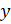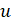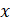DIFFERENTIATION II – APPLICATION OF DIFFERENATION

Mathematics SSS 3 First Term

WEEK 12

DIFFERENTIATION II – APPLICATION OF DIFFERENATION

Performance Objectives

Student should be able to:

1. Apply the rules of differentiation (function-of-function).
2. Apply differentiation in determining maximum and minimum point, acceleration, velocity and rate of change.

Content

Chain Rule for Derivative of a Composite Function

Supposeis a function of a variable, say, where uis a function of another variable, we then say that yis a function of a function of